# VBA Insert ColumnsArticle byExcelMojo Team## What Is Insert Column In Excel Using VBA?

VBA Insert Columns refers to adding or inserting new columns to the worksheet in Excel using VBA. As part of data manipulation, one must insert multiple columns at times. We use the right-click and Insert method in Excel to insert columns. However, in VBA, the task is more complex.

We must write an Insert command code and decide whether to VBA insert columns before, to the left, or the right. For example, look at the following data in Excel.

Assume we must insert a column between columns A and B. We use the following code to insert a column. Upon executing the VBA code, it will insert a new column between A and B, as shown below.

###### Key Takeaways
• Insert method will insert a new column by pushing the existing column to the right side.
• We can reference the column by using the RANGE object as well as COLUMNS property.
• We can insert alternative columns by using loops one after the other.
• We can insert columns based on the cell value by applying the logical IF condition.
• Whenever we reference a cell to insert a new column, only the Insert method will not work. Hence, we must use the “EntireColumn” property first, then use the Insert method.

### How to Insert Column in Excel Worksheet using VBA?

We can insert columns in Excel VBA using multiple methods. The common ones to be used to insert a column are as follows.

• Columns Property
• Range Object
• Cells Property

We can use any one of the above methods to insert a column. Using any one of the above methods depends on the requirement, and we can use them as an alternative to each other.

Let us show you a step-by-step approach to inserting a column. For example, look at the following data in Excel.

Assume we must insert a column after column B. Follow the below steps.

1. Create a sub-routine procedure by naming the macro.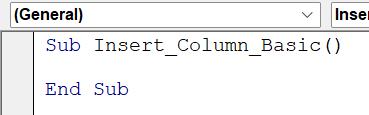2. Since we need to insert a column after column B, let’s reference column C using the COLUMNS property.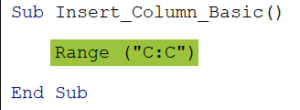3. Enter a dot to choose the I+nsert method once the column is referenced.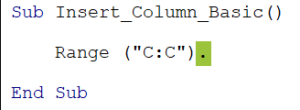When we enter the dot, we should have seen the IntelliSense list showing the properties and methods associated with the COLUMNS property. However, whenever we use the COLUMNS property, we do not see any IntelliSense list, which will be a setback for someone starting in Excel VBA.

Hence, using the RANGE object is always recommended, which allows us to see the IntelliSense list.

4. Let’s use the RANGE object and reference the entire column. We enter a dot to see the IntelliSense list, which shows the properties and methods associated with the RANGE object.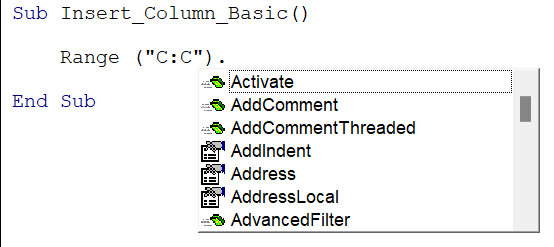5. Choose the Insert method from the list.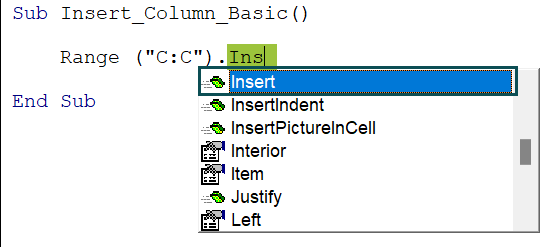6. Once the Insert method is chosen, we can see its arguments.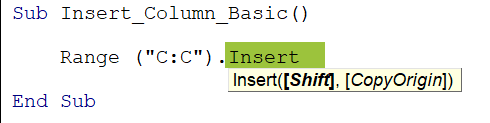7. We must use the “xlToRight” command for the argument Shift because the referenced column “C” should be moved to the right side.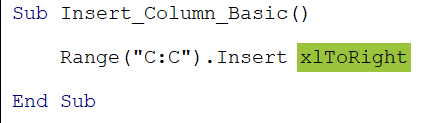Now, let us execute the code. It will insert a new column by pushing the existing column “C” to the right.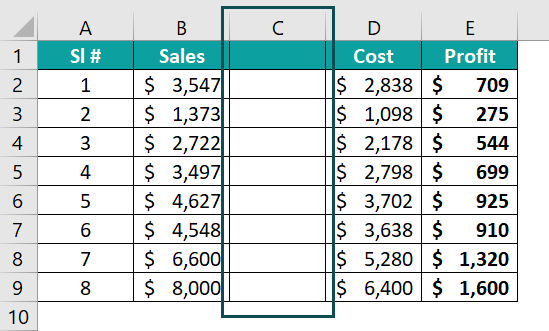### Examples of Insert Columns in Excel using VBA

Let us show you different scenarios of using the VBA Insert Columns method.

#### Example #1 – Insert Columns using Range Object in VBA

As we have seen in the previous example, we get access to the IntelliSense list whenever we use a RANGE object in VBA, which helps us construct the code. Similarly, let us look at another example.

Assume we must insert a column after the current column “E.” First, we must reference the column to be moved to the right when we wish to insert a new column.

Since we are inserting a new column after column “E,” we must reference the column “F.”

Once the column to be moved to the right is chosen, we have to select the Insert method.

The default value for the shift argument of the VBA insert columns method is “xlToRight.”

Sub Insert_Column_Ex1()
Range(“F:F”).Insert xlToRight
End Sub

Let’s execute the code. It will insert a new column by pushing the existing column “F” to the right side.

#### Example #2 – Insert Multiple Columns

We can also insert multiple columns in one go. For example, let’s use the same data from the previous example.

Assume we must insert three VBA insert columns to right after column “B” Hence, we must reference three columns after column “B,” i.e., Column “C,” “D,” and “E.”

We have given the starting and the ending columns. The column “D” is also included in this reference. Now, choose the Insert method.

Sub Insert_Column_Ex2()
Range(“C:E”).Insert
End Sub

When we execute this code, we will get multiple columns inserted.

All three columns carry the formatting from the left column, i.e., column “B.”

#### Example #3 – Insert Columns using COLUMNS Property in VBA

Instead of a RANGE object, we can use the COLUMNS property in VBA to insert columns. As discussed earlier, one of its drawbacks is that we do not get to see the IntelliSense list and must completely rely on VBA skills.

Assume we need to insert a column after column “C.” Here, we must reference the column by its numerical number when we use the COLUMNS property.

Sub Insert_Column_Ex3()
Columns(3).Insert
End Sub

Since column “C” is the third column in the columns list, we have referenced it using numerical 3. Once we execute this code, we will get the new column inserted.

The new column has been inserted after column 2 and the existing column 3 is pushed to column 4. It is important to be absolutely sure of the properties and methods associated with the VBA COLUMNS property when using it.

#### Example #4 – Insert Alternative Columns

It is not often that we insert alternative columns, but we may need to at times. Hence, it is necessary to know the technique of inserting blank columns after every alternative column.

For example, look at the following data.

Assume we must insert a new column after every alternative column, i.e., as shown below.

We can use the following code to insert alternative blank columns.

Sub Insert_Column_ex4()
‘Define a variable to find total number of columns
Dim LC As Long
‘Find total columns
LC = Cells(1, Columns.Count).End(xlToLeft).Column
‘Select the first column where we need to insert a new column
Columns(2).Select
‘Define a variable to find assign the loop
Dim k As Long
‘Initiate the loop
For k = 2 To LC
‘Insert a new column from the active cell
ActiveCell.EntireColumn.Insert
‘After inserting a new column move two cells to the right
ActiveCell.Offset(0, 2).Select
Next k
End Sub

Let’s execute this code and we will get the following result in a worksheet.

As seen, we have got new blank columns as alternative columns. This code is dynamic; we can have as many columns as possible.  It will dynamically insert blank columns after every column instead of VBA insert columns to left.

#### Example #5 – Insert Column Based on Cell Value

We can apply some logical conditions to insert a column. For example, if the cell header is equal to something then we can insert a new column or else we can simply move on to the next column.

For example, look at the following data in Excel.

We have employee-wise sales information for every 3 months. After three months, we have a name column repeated and this looks congested for the readers.

Let us insert a blank column before a column with names begins. We can use the following code to insert a column based on the condition.

Brief Explanation of the Code

• Part #1: Here, we have defined a variable to find the last used column, so we know how many columns we have in total.
• Part #2: We have defined a variable to start the column number. Initially it is set to 2, so that when the loop starts it will begin from column 2.
• Part #3: We have defined a variable which is to be used in the FOR loop.
• Part #4: We have initiated the loop. Inside the loop, we have applied an IF logical condition to check if the current looping column header value is equal to “Name.” If yes, then it will insert a new column in that current looping column or else it will exit the IF condition.

In this condition, we have applied a i = i + 1 to increase the column number by 1 when the loop runs each time. Let’s execute the code and we will get to see the following result in a worksheet.

As we can see wherever we have column header “Name” we can see the blank column before that column.

### Important Things to Note

• The IntelliSense list which displays properties and methods is not available if we use the COLUMNS property.
• Whenever we use COLUMNS property, we must give only the column number but do not use double quotes.
• Whenever we add a new column, the existing column by default will be pushed to the right side and all the subsequent columns which are to the right will move from their positions.
• By default, xlToRight is the insert option for VBA Insert Column method.

1. Why is insert columns in VBA not working

VBA Insert columns require correct reference of columns, if the reference of columns is invalid then it will not insert a column rather throws an error.

2. How to insert multiple columns to a ComboBox in VBA?

We cannot insert multiple columns to a ComboBox using the VBA Insert Columns method.

3. How do you insert columns to the right in Excel?

By default, whenever we insert a new column, the column will be inserted to the right because the default value for the Shift argument of the INSERT method is xlToRight.